## Binomial point process

The binomial point process is arguably the simplest point process. It consists of a non-random number of points scattered randomly and independently over some bounded region of space. In this post I will describe the binomial point process, how it leads to the Poisson point process, and its historical role as stars in the sky.

The binomial point process is an important stepping stone in the theory of point process. But I stress that for mathematical models, I would always use a Poisson point process instead of a binomial one. The only exception would be if you were developing a model for a small, non-random number of points.

## Uniform binomial point process

We start with the simplest binomial point process, which has uniformly located points. (I described simulating this point process in an early post. The code is here.)

### Definition

Consider some bounded (or more precisely, compact) region, say, $$W$$, of the plane plane $$\mathbb{R}^2$$, but the space can be more general.  The uniform binomial point process is created by scattering $$n$$ points uniformly and independently across the set $$W$$.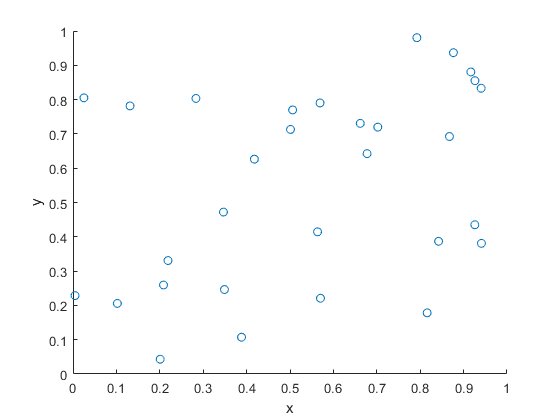A single realization of a binomial point process with n=30 points. The points are uniformly and independently scattered across a unit square.

### Distribution

Consider a single point uniformly scattered in the region $$W$$, giving a binomial point process with $$n=1$$.  We look at some region $$B$$, which is a subset of $$W$$, implying $$B\subseteq W$$.  What is the probability that the single point $$X$$ falls in region $$B$$?

First we write $$\nu(W)$$ and $$\nu(B)$$  to denote the respective areas (or more precisely, Lebesgue measures) of the regions $$W$$ and $$B$$, hence $$\nu(B)\leq \nu(W)$$. Then this probability, say, $$p$$, is simply the ratio of the two areas, giving

$$p= P(X\in B)=\frac{\nu(B)}{\nu(W)}.$$

The event of a single point being found in the set $$B$$ is a single Bernoulli trial, like flipping a single coin. But if there are $$n$$ points, then there are $$n$$ Bernoulli trials, which bring us to the binomial distribution.

For a uniform binomial point process $$N_W$$, the number of randomly located points being found in a region $$B$$ is a binomial random variable, say, $$N_W(B)$$, with probability parameter $$p=\nu(B)/ \nu(W)$$. The probability mass function of $$N_W(B)$$ is

$$P(N_W(B)=k)={n\choose k} p^k(1-p)^{n-k}.$$

We can write the expression more explicitly

$$P(N_W(B)=k)={n\choose k} \left[\frac{\nu(B)}{ \nu(W)}\right]^k\left[1-\frac{\nu(B)}{\nu(W)}\right]^{n-k}.$$

## Poisson limit

### Poisson random variable

A standard exercise in introductory probability is deriving the Poisson distribution by taking the limit of the binomial distribution. This is done by sending $$n$$ (the total number of Bernoulli trials) to infinity while keeping the binomial mean $$\mu:=p n$$ fixed, which sends the probability $$p=\mu/n$$ to zero.

More precisely, for $$\mu\geq0$$, setting $$p_n=\mu/n$$ and keeping $$\mu :=p_n n$$ fixed, we have the limit result

$$\lim_{n\to \infty} {n \choose k} p_n^k (1-p_n)^{n-k} = \frac{\mu^k}{k!}\, e^{-\mu}.$$

We can use, for example, Stirling’s approximation to prove this limit result.

We can make the same limit argument with the binomial point process.

### Homogeneous Poisson point process

We consider the intensity of the uniform binomial point process, which is the average number of points in a unit area. For a binomial point process, this is simply

$$\lambda := \frac{n}{\nu(W)}.$$

For the Poisson limit, we expand the region $$W$$ so it covers the whole plane $$\mathbb{R}^2$$, while keeping the intensity $$\lambda = n/\nu(W)$$ fixed. This means that the area $$\nu(W)$$ approaches infinity while the probability $$p=\nu(B)/\nu(W)$$ goes to zero. Then in the limit we arrive at the homogeneous Poisson point process $$N$$ with intensity $$\lambda$$.

The number of points of $$N$$ falling in the set $$B$$ is a random variable $$N(B)$$ with the probability mass function

$$P(N(B)=k)=\frac{[\lambda \nu(B)]^k}{k!}\,e^{-\lambda \nu(B)}.$$

## General binomial point process

Typically in point process literature, one first encounters the uniform binomial point process. But we can generalize it so the points are distributed according to some general distribution.

### Definition

We write $$\Lambda$$ to denote a non-negative Radon measure on $$W$$, meaning $$\Lambda(W)< \infty$$ and $$\Lambda(B)\geq 0$$ for all (measurable) sets $$B\subseteq W$$.  We can also assume a more general space for the underlying space such as a compact metric space, which is (Borel) measurable. But the intuition still works for compact region of the plane $$\mathbb{R}^2$$.

For the $$n$$ points, we assume each point is distributed according to the probability measure

$$\bar{\Lambda}= \frac{\Lambda}{\Lambda(W)}.$$

The resulting point process is a general binomial point process. The proofs for this point process remain essentially the same, replacing the Lebesgue measure $$\nu$$, such as area or volume, with the non-negative measure $$\Lambda$$.

### Example

A typical example of the intensity measure $$\Lambda$$ has the form

$$\Lambda(B)= \int_B f(x) dx\,,$$

where $$f$$ is a non-negative density function on $$W$$. Then the probability density of a single point is

$$p(x) = \frac{1}{c}f(x),$$

where $$c$$ is a normalization constant

$$c= \int_W f(x) dx\,.$$

On a set $$W \subseteq \mathbb{R}^2$$ using Cartesian coordinates, a specific example of the density $$f$$ is

$$f(x_1,x_2) = \lambda e^{-(x_1^2+x_2^2)}.$$

### Distribution

Assuming a general binomial point process $$N_W$$ on $$W$$, we can use the previous arguments to obtain the binomial distribution

$$P(N_W(B)=k)={n\choose k} \left[\frac{\Lambda(B)}{\Lambda(W)}\right]^k\left[1-\frac{\Lambda(B)}{\Lambda(W)}\right]^{n-k}.$$

### General Poisson point process

We can easily adapt the Poisson limit arguments for the general binomial Poisson point process, which results in the general Poisson point process $$N$$ with intensity measure $$\Lambda$$. The number of points of $$N$$ falling in the set $$B$$ is a random variable $$N(B)$$ with the probability mass function

$$P(N(B)=k)=\frac{[\Lambda(B)]^k}{k!}\, e^{-\Lambda(B)}.$$

## History: Stars in the sky

The uniform binomial point process is an example of a spatial point process. With points being scattered uniformly and independently, its sheer simplicity makes it a natural choice for an early spatial model. But which scattered objects?

Perhaps not surprisingly, it is trying to understand star locations where we find the earliest known example of somebody describing something like a random point process. In 1767 in England John Michell wrote:

what it is probable would have been the least apparent distance of any two or more stars, any where in the whole heavens, upon the supposition that they had been scattered by mere chance, as it might happen

As an example, Michelle studied the six brightest stars in the Pleiades star cluster. He concluded the stars were not scattered by mere chance. Of course “scattered by mere chance” is not very precise in today’s probability language, but we can make the reasonable assumption that Michell meant the points were uniformly and independently scattered.

Years later in 1860 Simon Newcomb  examined Michell’s problem, motivating him to derive the Poisson distribution as the limit of the binomial distribution. Newcomb also studied star locations. Stephen Stigler considers this as the first example of applying the Poisson distribution to real data, pre-dating the famous work by Ladislaus Bortkiewicz who studied rare events such as deaths from horse kicks. We also owe Bortkiewicz the terms Poisson distribution and stochastic (in the sense of random).

### Code

Here, on my repository, are some pieces of code that simulate a uniform binomial point process on a rectangle.

For an introduction to spatial statistics, I suggest the lectures notes by Baddeley, which form Chapter 1 of these published lectures, edited by Baddeley, Bárány, Schneider, and Weil. The binomial point process is covered in Section 1.3.

The binomial point process is also covered briefly in the classic text Stochastic Geometry and its Applications by Chiu, Stoyan, Kendall and Mecke; see Section 2.2. Similar material is covered in the book’s previous edition by Stoyan, Kendall and Mecke.

Haenggi also wrote a readable introductory book called Stochastic Geometry for Wireless networks, where he gives the basics of point process theory. The binomial point process is covered in Section 2.4.4.

For some history on point processes and the Poisson distribution, I suggest starting with the respective papers:

• Guttorp and Thorarinsdottir, What Happened to Discrete Chaos, the Quenouille Process, and the Sharp Markov Property?;
• Stigler, Poisson on the Poisson distribution.

Histories of the Poisson distribution and the Poisson point process are found in the books:

## Simulating Poisson random variables in Fortran

The hint’s in the title. I wrote a simple function in Fortran for simulating (or sampling) Poisson random variables. (More precisely, I should say that the function generates Poisson variates.) I used the simple direct method. This method is based on the exponential inter-arrival times of the Poisson (stochastic) process.

My code should not be used for large Poisson parameter values (larger than, say, 20 or 30), as the code may be too slow. Other methods exist for larger parameter values, which I’ve discussed previously.

I just use the standard Fortran function random_number for generating (pseudo-)random numbers. I am not an expert in Fortran, but my Poisson function seems to work fine. I wrote and ran a simple test that estimates the first and second moments, which should match for Poisson variables.

My Fortran code is very similar to the code that I wrote in C and C#, which is located here. You should be able to run it on this website or similar ones that can compile Fortran (95) code.

For various Poisson simulation methods, see the stochastic simulation books by Devroye (Section X.3) or Fishman (Section 8.16). There’s a free online version of Devroye’s book here. The book by Gentle (Section 5.2.8) also briefly covers Poisson variables.

In this post on generating Poisson variates, John D. Cook briefly discusses the direct method (for small Poisson parameter values), as well as a rejection method from a 1979 paper by Atkinson.

I wrote the Poisson code using Fortran 95. There are various books and websites on Fortran. The website tutorialspoint.com gives a good introduction to Fortran. You can also edit, compile and run your Fortran code there with its online Fortran editor. I found this short summary a good start. For alternative Fortran code of a Poisson generator, consult the classic book Numerical Recipes, though I believe the book versions only exist for Fortran 77 and Fortran 90.

## Code

On this page I’ve only included the code of the functions for generating uniform and Poisson variates. The rest of the code, including the test, is located here.

!Poisson function -- returns a single Poisson random variable
function funPoissonSingle(lambda) result(randPoisson)
real, intent(in) :: lambda !input
real :: exp_lambda !constant for terminating loop
real :: randUni !uniform variable
real :: prodUni !product of uniform variables
integer :: randPoisson !Poisson variable

exp_lambda= exp(-lambda)

!initialize variables
randPoisson = -1;
prodUni = 1;
do while (prodUni > exp_lambda)
randUni = funUniformSingle() !generate uniform variable
prodUni = prodUni * randUni; !update product
randPoisson = randPoisson + 1 !increase Poisson variable
end do
end function

!Uniform function -- returns a standard uniform random variable
function funUniformSingle() result(randUni)
real randUni;
call random_seed
call random_number(randUni)

end function


## Simulating a Poisson point process on a circle

In this post, I’ll take a break from the more theoretical posts. Instead I’ll describe how to simulate or sample a homogeneous Poisson point process on a circle.  I have already simulated this point process on a rectangle, triangle and disk. In some sense, I should have done this simulation method before the disk one, as it’s easier to simulate. I recommend reading that post first, as the material presented here builds off it.

Sampling a homogeneous Poisson point process on a circle is rather straightforward.  It just requires using a fixed radius and uniformly choose angles from interval $$(0, 2\pi)$$. But the circle setting gives an opportunity to employ a different method for positioning points uniformly on circles and, more generally, spheres. This approach uses Gaussian random variables, and it becomes much more efficient when the points are placed on high dimensional spheres.

## Steps

Simulating a Poisson point process requires two steps: simulating the random number of points and then randomly positioning each point.

##### Number of points

The number of points of a Poisson point process on circle of radius $$r>0$$ is a Poisson random variable with mean $$\lambda C$$, where $$C=2\pi r$$ is the circumference of the circle.  You just need to be able to need to produce (pseudo-)random numbers according to a Poisson distribution.

To generate Poisson variables in MATLAB,  use the poissrnd function with the argument $$\lambda C$$.  In Python, use either the scipy.stats.poisson or numpy.random.poisson function from the SciPy or NumPy libraries. (If you’re curious how Poisson simulation works, I suggest seeing this post for details on sampling Poisson random variables or, more accurately, variates.)

### Locations of points

For a homogeneous Poisson point process, we need to uniformly position points on the underlying space, which is this case is a circle. We will look at two different ways to position points uniformly on a circle. The first is arguably the most natural approach.

##### Method 1: Polar coordinates

We use polar coordinates due to the nature of the problem. To position all the points uniformly on a circle, we simple generate uniform numbers on the unit interval $$(0,1)$$. We then multiply these random numbers by $$2\pi$$.

We have generated polar coordinates for points uniformly located on the circle. To plot the points, we have to convert the coordinates back to Cartesian form by using the change of coordinates:  $$x=\rho\cos(\theta)$$ and $$y=\rho\sin(\theta)$$.

##### Method 2: Normal random variables

For each point, we generate two standard normal or Gaussian random variables, say, $$W_x$$ and $$W_y$$, which are independent of each other. (The term standard here means the normal random variables have mean $$\mu =0$$ and standard deviation $$\sigma=1$$.) These two random variables are the Cartesian components of a random point.  We then rescale the two values by the Euclidean norm, giving

$$X=\frac{W_x}{(W_x^2+W_y^2)^{1/2}},$$

$$Y=\frac{W_y}{(W_x^2+W_y^2)^{1/2}}.$$

These are the Cartesian coordinates of points uniformly scattered around a unit circle with centre at the origin. We multiply the two random values $$X$$ and $$Y$$ by the $$r>0$$  for a circle with radius $$r$$.

###### How does it work?

The procedure is somewhat like the Box-Muller transform in reverse. I’ll give an outline of the proof. The joint density of the random variables $$W_x$$ and $$W_y$$ is that of the bivariate normal distribution with zero correlation, meaning it has the joint density

$$f(x,y)=\frac{1}{2\pi}e^{[-(x^2+y^2)/2]}.$$

We see that the function $$f$$ is a constant when we trace around any line for which $$(x^2+y^2)$$ is a constant, which is simply the Cartesian equation for a circle (where the radius is the square root of the aforementioned constant). This means that the angle of the point $$(W_x, W_y)$$ will be uniformly distributed.

Now we just need to look at the distance of the random point. The vector formed from the pair of normal variables $$(W_x, W_y)$$ is a Rayleigh random variable.  We can see that the vector from the origin to the point $$(X,Y)$$ has length one, because we rescaled it with the Euclidean norm.

## Results

I have presented some results produced by code written in MATLAB and Python. The blue points are the Poisson points on the sphere. I have used a surface plot (with clear faces) in black to illustrate the underling sphere.

##### MATLAB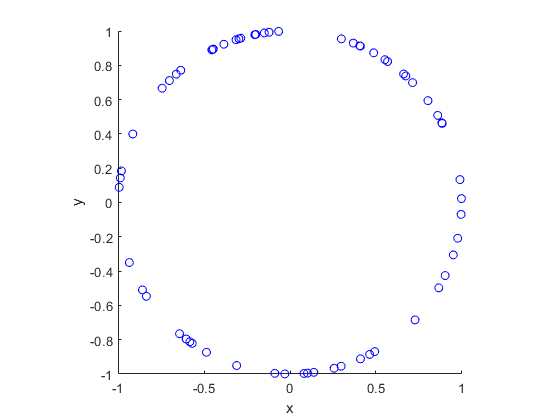##### Python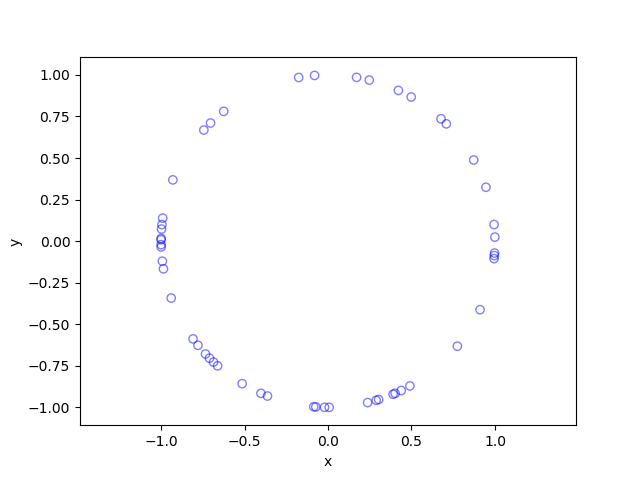## Code

The code for all my posts is located online here. For this post, the code in MATLAB and Python is here.

I recommend this blog post, which discusses different methods for randomly placing points on spheres and inside spheres (or, rather, balls) in a uniform manner.  (Embedded in two dimensions, a sphere is a circle and a ball is a disk.) A key paper on using normal variables is the following:

• 1959, Muller, A note on a method for generating points uniformly on n-dimensional spheres.

As I mentioned in the post on the disk, the third edition of the classic book Stochastic Geometry and its Applications by Chiu, Stoyan, Kendall and Mecke details on page 54 how to uniformly place points on a disk.  It just requires a small modification for the circle.

## Placing a random point uniformly in a Voronoi cell

In the previous post, I discussed how Voronoi or Dirichlet tesselations are useful and how they can be calculated or estimated with many scientific programming languages by using standard libraries usually based on Qhull. The cells of such a tessellation divide the underlying space. Now I want to randomly place a single point in a uniform manner in each bounded Voronoi cell.

But why?

Well, this task arises occasionally, particularly when developing mathematical models of wireless networks, such as mobile or cellular phone networks. A former colleague of mine had to do this, which inspired me to write some MATLAB code a couple of years ago. And I’ve seen the question posed a couple of times on the web . So I thought: I can do that.

## Overview

For this problem, I see two obvious methods.

##### Simple but crude

The simplest method is to cover each Voronoi cell with a rectangle or disk. Then randomly place a point uniformly on the rectangle or disk. If it doesn’t randomly land inside the rectangle or disk, then do it again until it does. Crude, slightly inefficient, but simple.

##### Elegant but slightly tricky

A more elegant way, which I will implement, is to partition (or divide) each Voronoi cell into triangles. Then randomly choose a triangle based on its area and, finally, uniformly position a point on that triangle.

## Method

##### Partition cells into triangles

It is straightforward to divide a Voronoi cell into triangles. Each side of the cell corresponds to one side of a triangle (that is, two points). The third point of the triangle is the original point corresponding to the Voronoi cell.

##### Choose a triangle

Randomly choosing a triangle is also easy. For a given cell, number the triangles. Which random triangle is chosen is simply a discrete random variable whose probability mass function is formed from triangle areas normalized (or divided) by the total area of the Voronoi cell. In other words, the probability of randomly choosing triangle $$i$$ with area $$A_i$$ from $$m$$ triangles is simply

$$P_i=\frac{A_i}{\sum_{j=1}^m A_j}.$$

To calculate the area of the triangles, I use the shoelace formula , which for a triangle with corners labelled $$\textbf{A}$$, $$\textbf{B}$$ and $$\textbf{C}$$ gives

$$A= \frac{1}{2} |(x_{\textbf{B}}-x_{\textbf{A}})(y_{\textbf{C}}-y_{\textbf{A}})-(x_{\textbf{C}}-x_{\textbf{A}})(y_{\textbf{B}}-y_{\textbf{A}})|.$$

But you can also use Herron’s formula.

Then the random variable is sampled using the probabilities based on the triangle areas.

##### Place point on chosen triangle

Given a triangle, the final step is also easy, if you know how, which is often the case in mathematics. I have already covered this step in a previous post, but I’ll give some details here.

To position a single point uniformly in the triangle, generate two random uniform variables on the unit interval $$(0,1)$$, say $$U$$ and $$V$$. The random $$X$$ and $$Y$$ coordinates of the single point are given by the expressions:

$$X=\sqrt{U} x_{\textbf{A}}+\sqrt{U}(1-V x_{\textbf{B}})+\sqrt{U}V x_{\textbf{C}}$$

$$Y=\sqrt{U} y_{\textbf{A}}+\sqrt{U}(1-V y_{\textbf{B}})+\sqrt{U}V y_{\textbf{C}}$$

## Results

The blue points are the points of the underlying point pattern that was used to generate the Voronoi tesselation. (These points have also been arbitrarily numbered.) The red points are the random points that have been uniformly placed in all the bounded Voronoi cells.

##### MATLAB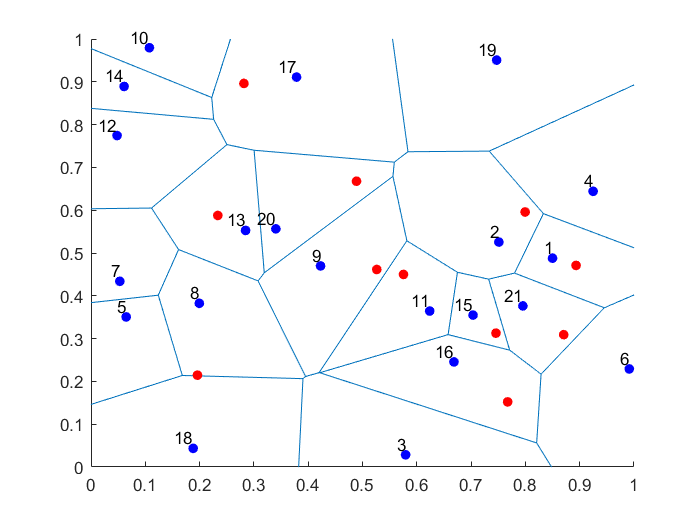##### Python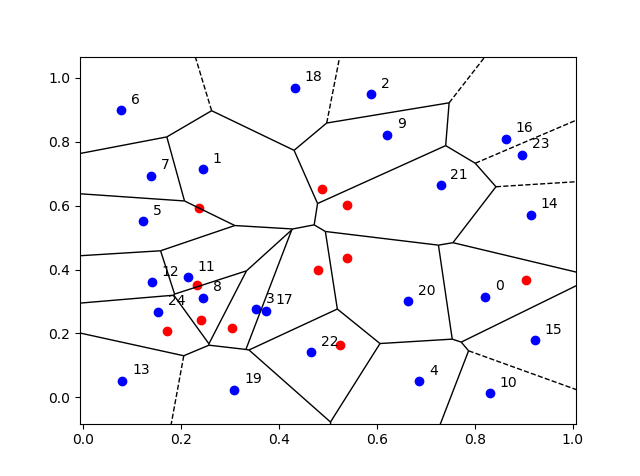## Empirical validation

We can empirically validate that the points are being placed uniformly on the bounded Voronoi cells. For a given (that is, non-random) Voronoi cell, we can repeatedly place (or sample) a random point uniformly in the cell. Increasing the number of randomly placed points, the respective averages of the $$x$$ and $$y$$ coordinates of the points will converge to the centroids (or geometric centres) of the Voronoi cell, which can be calculated with simple formulas.

## Code

The code for all my posts is located online here. For this post, the code in MATLAB and Python is here.

I have also written in MATLAB and Python the code as functions (in files funVoronoiUniform.m and funVoronoiUniform.py, respectively), so people can use it more easily. The function files are located here, where I have also included an implementation of the aforementioned empirical test involving centroids. You should be able to use those functions and for any two-dimensional point patterns.

Much has been written on Voronoi or Dirichlet tessellations, particularly when the seeds of the cells form a Poisson point process. For references, I recommend my previous post, where I say that the references in the articles on Wikipedia and MathWorld are good starting points.

In this StackExchange post, people discuss how to place a single point uniformly on a general triangle. For the formulas, the thread cites the paper Shape distributions by Osada, Funkhouser, Chazelle and Dobkin, where no proof is given.

## Simulating a Cox point process based on a Poisson line process

In the previous post, I described how to simulate a Poisson line process, which in turn was done by using insight from an earlier post on the Bertrand paradox.

Now, given a Poisson line process, for each line, if we generate an independent one-dimensional Poisson point point process on each line, then we obtain an example of a Cox point process. Cox point processes are also known as doubly stochastic Poisson point processes. On the topic of names, Guttorp and Thorarinsdottir argue that it should be called the Quenouille point process, as Maurice Quenouille introduced an example of it before Sir David Cox, but I opt for the more common name.

## Cox point proceesses

A Cox point process is a generalization of a Poisson point process. It is created by first considering a non-negative random measure, sometimes called a driving measure. Then a Poisson point process, which is independent of the random driving measure, is generated by using the random measure as its intensity or mean measure.

The driving measure of a Cox point process can be, for example, a non-negative random variable or field multiplied by a Lebesgue measure. In our case, the random measure is the underlying Poisson line process coupled with the Lebesgue measure on the line (that is, length).

Cox processes form a very large and general family of point processes, which exhibit clustering. In previous posts, I have covered two special cases of Cox point processes: the Matérn and Thomas cluster point processes. These are, more specifically, examples of a Neyman-Scott point process, which is a special case of a shot noise Cox point process. These two point processes are fairly easy to simulate, but that’s not the case for Cox point processes in general. Some are considerably easier than others.

## Motivation

I will focus on simulating the Cox point process formed from a Poisson line process with homogeneous Poisson point processes. I do this for two reasons. First, it’s easy to simulate, given we can simulate a Poisson line process. Second, it has been used and studied recently in the mathematics and engineering literature for investigating wireless communication networks in cities, where the streets correspond to Poisson lines; for example, see these two preprints:

Incidentally, I don’t know what to call this particular Cox point process. A Cox line-point process? A Cox-Poisson line-point process? But it doesn’t matter for simulation purposes.

## Method

We will simulate the Cox (-Poisson line-) point process on a disk. Why a disk? I suggest reading the previous posts on the Poisson line process the Bertrand paradox for why the disk is a natural simulation window for line processes.

Provided we can simulate a Poisson line process, the simulation method is quite straightforward, as I have essentially already described it.

##### Line process

First simulate a Poisson line process on a disk. We recall that for each line of the line process, we need to generate two independent random variables $$\Theta$$ and $$P$$ describing the position of the line. The first random variable $$\Theta$$ gives the line orientation, and it is a uniform random variable on the interval $$(0,2\pi)$$.

The second random variables $$P$$ gives the distance from the origin to the disk edge, and it is a uniform random variable on the interval $$(0,r)$$, where $$r$$ is the radius of the disk. The distance from the point $$(\Theta, P)$$ to the disk edge (that is, the circle) along the chord is:

$$Q=\sqrt{r^2-P^2}.$$

The endpoints of the chord (that is, the points on the disk edge) are then:

Point 1: $$X_1=P \cos \Theta+ Q\sin \Theta$$, $$Y_1= P \sin \Theta- Q\cos \Theta$$,

Point 2: $$X_2=P \cos \Theta- Q\sin \Theta$$, $$Y_2= P \sin \Theta+Q \cos \Theta$$.

The length of the line segment is $$2 Q$$. We can say this random line is described by the point $$(\Theta,P)$$.

##### One-dimensional Poisson point process

For each line (segment) in the line process, simulate a one-dimensional Poisson point process on it. Although I have never discussed how to simulate a one-dimensional (homogeneous) Poisson point process, it’s essentially one dimension less than simulating a homogeneous Poisson point process on a rectangle.

More specifically, given a line segment $$(\Theta,P)=(\theta,p)$$, you simulate a homogeneous Poisson point process with intensity $$\mu$$ on a line segment with length $$2 q$$, where $$q=\sqrt{r^2-p^2}$$. (I am now using lowercase letters to stress that the line is no longer random.) To simulate the homogeneous Poisson point process, you generate a Poisson random variable with parameter $$2 \mu q$$.

Now you need to place the points uniformly on the line segment. To do this, consider a single point on a single line. For this point, generate a single uniform variable $$U$$ on the interval $$(-1,1)$$. The tricky part is now getting the Cartesian coordinates right. But the above expressions for the endpoints suggest that the single random point has the Cartesian coordinates:

$$x=p \cos \theta+ U q\sin \theta$$, $$y=p \sin \theta- U q\cos \theta$$.

The two extreme cases of the uniform random variable $$U$$ (that is, $$U=-1$$ and $$U=1$$) correspond to the two endpoints of the line segment. We recall that $$Q$$ is the distance from the midpoint of the line segment to the disk edge along the line segment, so it makes sense that we want to vary this distance uniformly in order to uniformly place a point on the line segment. This uniform placement step is done for all the points of the homogeneous Point process on that line segment.

You repeat this procedure for every line segment. And that’s it: a Cox point process built upon a Poisson line process.

## Results

##### MATLAB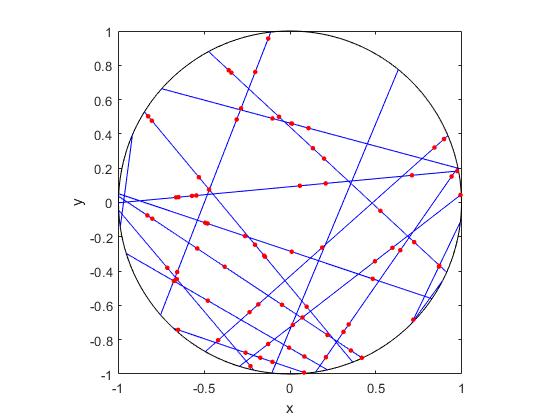##### R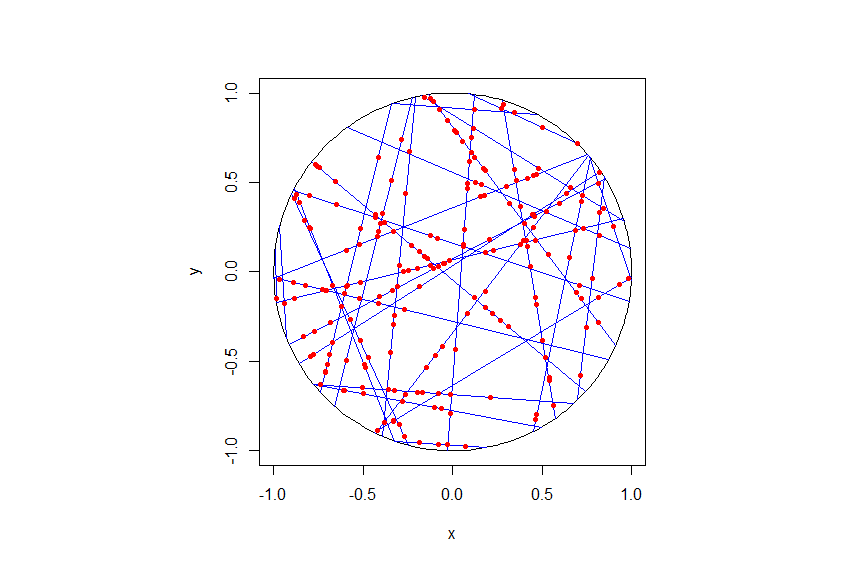##### Python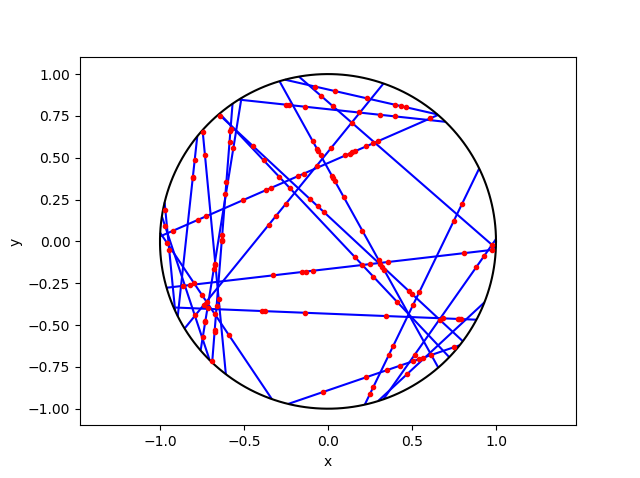## Code

As always, the code from all my posts is online. For this post, I have written the code in MATLAB, R and Python.

For the first step, the reading material is basically the same as that for the Poisson line process, which overlaps with that of the Bertrand paradox. For the one-dimensional Poisson point process, we can use the reading material on the homogeneous Poisson point process on a rectangle.

For general Cox point processes, I recommend starting with the following: Chapter 6 in the monograph Poisson Processes by Kingman; Chapter 5 in Statistical Inference and Simulation for Spatial Point Processes by Møller and Waagepetersen; and Section 5.2 in Stochastic Geometry and its Applications by Chiu, Stoyan, Kendall and Mecke. For a much more mathematical treatment, see Chapter 13 in Lectures on the Poisson Process by Last and Penrose, which is freely available online here.

For this particularly Cox point process, see the two aforementioned preprints, located here and here.

## Simulating a Poisson line process

Instead of points, we can consider other objects randomly scattered on some underlying mathematical space. If we take a Poisson point process, then we can use (infinitely long) straight lines instead of points, giving a Poisson line process. Researchers have studied and used this random object to model physical phenomena. In this post I’ll cover how to simulate a homogeneous Poisson line process in MATLAB, R and Python. The code can be downloaded from here

## Overview

For simulating a Poisson line process, the key question is how to randomly position the lines.  This is related to a classic problem in probability theory called the Bertrand paradox.  I discussed this illustration in probability in a previous post, where I also included code for simulating it. I highly recommend reading that post first.

The Bertrand paradox involves three methods for positioning random lines. We use Method 2 to achieve a uniform positioning of lines, meaning the number of lines and orientation of lines is statistically uniform. Then it also makes sense to use this method for simulating a homogeneous (or uniform) Poisson line process.

We can interpret a line process as a point process. For a line process on the plane $$\textbf{R}^2$$, it can be described by a point process on $$(0,\infty)\times (0,2\pi)$$, which is an an infinitely long cylinder. In other words, the Poisson line process can be described as a Poisson point process.

For simulating a Poisson line process, it turns out the disk is the most natural setting. (Again, this goes back to the Bertrand paradox.) More specifically, how the (infinitely long) lines intersect a disk of a fixed radius $$r>0$$. The problem of simulating a Poisson line process reduces to randomly placing chords in a disk. For other simulation windows in the plane, we can always bound any non-circular region with a sufficiently large disk.

## Steps

##### Number of lines

Of course, with all things Poisson, the number of lines will be  a Poisson random variable, but what will its parameter be? This homogeneous (or uniform) Poisson line process forms a one-dimensional homogeneous (or uniform) Poisson point process around the edge of the disk with a circumference $$2 \pi r$$. Then the number of lines is simply a Poisson variable with parameter $$\lambda 2 \pi r$$.

##### Locations of points

To position a single line uniformly in a disk, we need to generate two uniform random variables. One random variable gives the angle describing orientation of the line, so it’s a uniform random variable $$\Theta$$ on the interval $$(0,2\pi)$$.

The other random variable gives the distance from the origin to the disk edge, meaning it’s a uniform random variable $$P$$ on the interval $$(0,r)$$, where $$r$$ is the radius of the disk.  The random radius and its perpendicular chord create a right-angle triangle.  The distance from the point $$(\Theta, P)$$ to the disk edge (that is, the circle) along the chord is:

$$Q=\sqrt{r^2-P^2}.$$

The endpoints of the chord (that is, the points on the disk edge) are then:

Point 1: $$X_1=P \cos \Theta+ Q\sin \Theta$$, $$Y_1= P \sin \Theta- Q\cos \Theta$$,

Point 2: $$X_2=P \cos \Theta- Q\sin \Theta$$, $$Y_2= P \sin \Theta+Q \cos \Theta$$.

## Code

I have implemented the simulation procedure in MATLAB, R and Python, which, as usual, are all very similar. I haven’t put my code here, because the software behind my website keeps mangling it.  As always, I have uploaded my code to a repository; for this post, it’s in this directory.

I have written the code in R, but I wouldn’t use it in general. That’s because if you’re using R, then, as I have said before, I strongly recommend using the powerful spatial statistics library spatstat. For a simulating Poisson line process, there’s the function rpoisline.

The chief author of spatstat, Adrian Baddeley, has written various lectures and books on the related topics of point processes, spatial statistics, and geometric probability. In this post, he answered why the angular coordinates have to be chosen uniformly.

### Results

MATLAB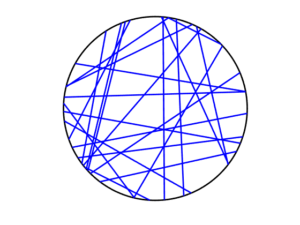R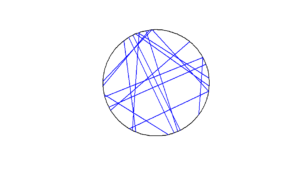Python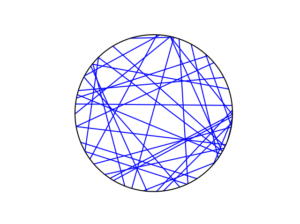To read about the Poisson line process, it’s best to start with the Bertrand problem, which is covered in many works on geometric probability and related fields. A good and recent introduction is given by Calka in (Section 1.3) of the lectures titled Stochastic Geometry: Modern Research Frontiers, which were edited by Coupier and published by Springer.  Also see, for example, problem 1.2 in Geometrical Probability by Kendall and Moran or page 44 in Geometric Probability by Solomon.

For the Poisson line process, I highly recommend Section 7.2 in the monograph Poisson Processes by Kingman. Also see Example 8.2 in the standard textbook Stochastic Geometry and its Applications by Chiu, Stoyan, Kendall and Mecke. The Poisson line process book also appears in Exercise 5.2 in the book Stochastic Simulation – Algorithms and Analysis by Asmussen and Glynn.

For online resources, this set of lectures by Christian Lantuéjoul
covers the Poisson line process. Wilfrid Kendall covers the Poisson line process in this talk in relation to so-called Poisson cities.

Mathematical paradoxes are results or observations in mathematics that are (seemingly) conflicting, unintuitive, incomprehensible, or just plain bizarre. They come in different flavours, such as those that play with notions of infinity, which means they often make little or no sense in a physical world. Other paradoxes, particularly those in probability, serve as a lesson that the problem needs to be posed in a precise manner. The Bertrand paradox is one of these.

Joseph Bertrand posed the original problem in his 1889 book Calcul des probabilités, which is available online (albeit in French). It’s a great illustrative problem involving simple probability and geometry, so it often appears in literature on the (closely related) mathematical fields of geometric probability and integral geometry.

Based on constructing a random chord in a circle, the paradox involves a single mathematical problem with three reasonable but different solutions. It’s less a paradox and more a cautionary tale. It’s really asking the question: What exactly do you mean by random?

Consequently, over the years the Bertrand paradox has inspired debate, with papers arguing what the true solution is. I recently discovered it has even inspired some passionate remarks on the internet; read the comments at www.bertrands-paradox.com.

But I am less interested in the different interpretations or philosophies of the problem. Rather, I want to simulate the three solutions. This is not very difficult, provided some trigonometry and knowledge from a previous post, where I describe how to simulate a (homogeneous) Poisson point process on the disk.

I won’t try to give a thorough analysis of the solutions, as there are much better websites doing that. For example, this MIT website gives a colourful explanation with pizza and fire-breathing monsters. The Wikipedia article also gives a detailed though less creative explanation for the three solutions.

My final code in MATLAB, R and Python code is located here.

### The Problem

Bertrand considered a circle with an equilateral triangle inscribed it. If a chord in the circle is randomly chosen, what is the probability that the chord is longer than a side of the equilateral triangle?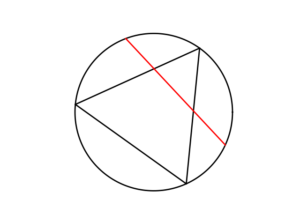### The Solution(s)

Bertrand argued that there are three natural but different methods to randomly choose a chord, giving three distinct answers. (Of course, there are other methods, but these are arguably not the natural ones that first come to mind.)

##### Method 1: Random endpoints

On the circumference of the circle two points are randomly (that is, uniformly and independently) chosen, which are then used as the two endpoints of the chord.

The probability of this random chord being longer than a side of the triangle is one third.

A radius of the circle is randomly chosen (so the angle is chosen uniformly), then a point is randomly (also uniformly) chosen along the radius, and then a chord is constructed at this point so it is perpendicular to the radius.

The probability of this random chord being longer than a side of the triangle is one half.

##### Method 3: Random midpoint

A point is randomly (so uniformly) chosen in the circle, which is used as the midpoint of the chord, and the chord is randomly (also uniformly) rotated.

The probability of this random chord being longer than a side of the triangle is one quarter.

### Simulation

All three answers involve randomly and independently sampling two random variables, and then doing some simple trigonometry. The setting naturally inspires the use of polar coordinates. I assume the circle has a radius $$r$$ and a centre at the point origin $$o$$. I’ll number the end points one and two.

In all three solutions we need to generate uniform random variables on the interval $$(0, 2\pi)$$ to simulate random angles. I have already done this a couple of times in previous posts such as this one.

##### Method 1: Random endpoints

This is probably the most straightforward solution to simulate. We just need to simulate two uniform random variables $$\Theta_1$$ and $$\Theta_2$$ on the interval $$(0, 2\pi)$$ to describe the angles of the two points.

The end points of the chord (in Cartesian coordinates) are then simply:

Point 1: $$X_1=r \cos \Theta_1$$, $$Y_1=r \sin \Theta_1$$,

Point 2: $$X_2=r \cos \Theta_2$$, $$Y_2=r \sin \Theta_2$$.

This method also involves generating two uniform random variables. One random variable $$\Theta$$ is for the angle, while the other $$P$$ is the random radius, which means generating the random variable $$P$$ on the interval $$(0, r)$$.

I won’t go into the trigonometry, but the random radius and its perpendicular chord create a right-angle triangle. The distance from the point $$(\Theta, P)$$ to the circle along the chord is:

$$Q=\sqrt{r^2-P^2}.$$

The endpoints of the chord are then:

Point 1: $$X_1=P \cos \Theta+ Q\sin \Theta$$, $$Y_1= P \sin \Theta- Q\cos \Theta$$,

Point 2: $$X_2=P \cos \Theta- Q\sin \Theta$$, $$Y_2= P \sin \Theta+Q \cos \Theta$$.

Take note of the signs in these expressions.

##### Method 3: Random midpoint

This method requires placing a point uniformly on a disk, which is also done when simulating a homogeneous Poisson point process on a disk, and requires two random variables $$\Theta’$$ and $$P’$$. Again, the angular random variable $$\Theta’$$ is uniform.

The other random variable $$P’$$ is not uniform. For $$P’$$, we generate a random uniform variable on the unit interval $$(0,1)$$, and then we take the square root of it. We then multiply it by the radius, generating a random variable between $$0$$ and $$r$$. (We must take the square root because the area element of a sector is proportional to the radius squared, and not the radius.) The distribution of this random variable is an example of the triangular distribution.

The same trigonometry from Method 2 applies here, which gives the endpoints of the chord as:

Point 1: $$X_1=P’ \cos \Theta’+ Q’\sin \Theta’$$, $$Y_1= P’ \sin \Theta’- Q’\cos \Theta’$$,

Point 2: $$X_2=P’\cos \Theta’- Q’\sin \Theta’$$, $$Y_2= P’\sin \Theta’+Q’ \cos \Theta’$$,

where $$Q’:=\sqrt{r^2-{P’}^2}$$. Again, take note of the signs in these expressions.

### Results

To illustrate how the three solutions are different, I’ve plotted a hundred random line segments and their midpoints side by side. Similar plots are in the Wikipedia article.

#####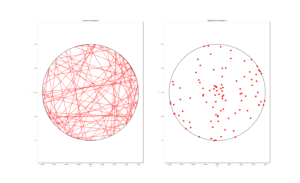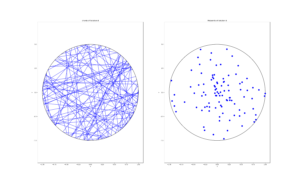##### Method 3: Random midpoint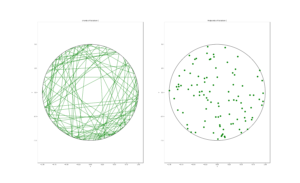### Conclusion

For the chord midpoints, we know and can see that Method 3 gives uniform points, while Method 2 has a concentration of midpoints around the circle centre. Method 1 gives results that seem to somewhere between Method 2 and 3 in terms of clustering around the circle centre.

For the chords, we see that Method 3 results in fewer chords passing through the circle centre. Methods 1 and 2 seem to give a similar number of lines passing through this central region.

It’s perhaps hard to see, but it can be shown that Method 2 gives the most uniform results. By this, I mean that the number of lines and their orientations statistically do not vary in different regions of the circle.

We can now position random lines in uniform manner. All we need now is a Poisson number of lines to generate something known as a Poisson line process, which will be the focus of the next post.

I’ve already mentioned that there are some good websites on the topic of the Bertrand paradox. For example:

web.mit.edu/tee/www/bertrand

www.cut-the-knot.org/bertrand.shtml

mathworld.wolfram.com/BertrandsProblem.html

Various authors have mentioned or discussed the Bertrand paradox in books on the related subjects of geometric probability, integral geometry and stochastic geometry. A good and recent introduction is given by Calka in Section 1.3 of the published lectures Stochastic Geometry: Modern Research Frontiers.

Other classic books that cover the topic including, for example, see Problem 1.2 in Geometrical Probability by Kendall and Moran. (Despite Maurice G. Kendall writing a book on geometric probability, he was not related to stochastic geometry pioneer David G Kendall.) It’s also discussed on page 44 in Geometric Probability by Solomon. For a book that involves more advance knowledge of geometry and (abstract) algebra, see Chapter 3 in Integral Geometry and Geometric Probability by Santaló.

The Bertrand paradox is also in The Pleasures of Probability by Isaac. It’s covered in a non-mathematical way in the book Paradoxes from A to Z by Clark. Edwin Jaynes studied the problem and proposed a solution in a somewhat famous 1973 paper, titled The Well-Posed Problem.

The original problem can be read in French in Bertrand’s work, which is available online here or here (starting at the bottom of page 4).

### Code

The MATLAB, R and Python code can be found here. In the code, I have labelled the methods A, B and C instead 1, 2 and 3.

## Point process simulation

Point processes are mathematical objects that can represent a collection of randomly located points on some underlying space. There is a rich range of these random objects, and, depending on the type of points process, there are various steps and methods used to sample, simulate or generate them on computers. (I use these three terms somewhat interchangeably.) This is the first of a series of posts in which I will describe how to simulate some of the more tractable point processes defined on bounded regions of two-dimensional Euclidean space.

### Finite point processes

A general family of point processes are the finite point processes, which are, as the name suggests, simply point processes with the property that the total number of points is finite with probability one. Usually it is assumed that both the number of points and the positions of these points are random. These two properties give a natural way to describe and to study point processes mathematically, while also giving an intuitive way to simulate them on computers.

If the number and locations of points can be simulated sequentially, such that the number of points is naturally generated first followed by the placing of the points, then the simulation process is usually easier. Such a point process is a good place to start for an example.

### Simple example — Binomial point process

The binomial point process is arguably the simplest point process. It is created by scattering $$n$$ points uniformly and independently located in some bounded region, say, $$R$$ with area $$|R|$$. The number of points located in a region $$B\subset R$$ is a binomial random variable, say, $$N$$, where its probability parameter $$p=\frac{|B|}{|R|}$$ is simply the ratio of the  two areas. This implies that

$$P(N=k)={n\choose k} p^k(1-p)^{n-k},$$

The total number of points is non-random number $$n$$, so we do not need to generate it as it is given. The independence of point locations means that all the points can be positioned in parallel.

For example, to simulate $$n$$ points of a binomial point process on the unit square $$[0,1]\times[0,1]$$, we just need to independently sample the $$x$$ and $$y$$ coordinates of the points from a uniform distribution on the unit interval $$[0,1]$$. In other words, we randomly generate or sample two sets of $$n$$ uniform random variables corresponding to the $$x$$ and $$y$$ of the $$n$$ points.

To sample on a general $$w \times h$$ rectangle, we just need need to multiple the random $$x$$ and $$y$$ values by the respective dimensions of the rectangle $$w$$ and $$h$$. Of course the rectangle can also be shifted up or down by adding or subtracting $$x$$ and $$y$$ values appropriately.

Essentially every programming language has a function for generating uniform random numbers because it is the default random number generator, which all the other random number generators build off, by employing various techniques like applying transformations. In MATLAB, R and Python, it is respectively rand, runif and scipy.stats.uniform, which all generate uniform points on the open interval $$(0,1)$$.

### Code

Here are some pieces of code that illustrates how to simulate a binomial point process on the unit square. I suggest downloading the code directly here from my repository. (Sometimes my webpage editor mangles simulation code.)

MATLAB

n=10; %number of points

%Simulate binomial point process
xx=rand(n,1);%x coordinates of binomial points
yy=rand(n,1);%y coordinates of binomial points

%Plotting
scatter(xx,yy);
xlabel('x');ylabel('y');


R

n=10; #number of points
#Simulate Binomial point process
xx=runif(n);#x coordinates of binomial points
yy=runif(n);#y coordinates of binomial points

#Plotting
plot(xx,yy,'p',xlab='x',ylab='y',col='blue');


Python

&lt;/pre&gt;
import numpy as np
import matplotlib.pyplot as plt

plt.close('all'); # close all figures

numbPoints = 10; # number of points

# Simulate binomial point process
xx = np.random.uniform(0, 1, numbPoints) # x coordinates of binomial points
yy = np.random.uniform(0, 1, numbPoints) # y coordinates of binomial points

# Plotting
plt.scatter(xx, yy, edgecolor='b', facecolor='none', alpha=0.5)
plt.xlabel('x')
plt.ylabel('y')
plt.show()
&lt;pre&gt;

### More complicated point processes

The binomial point process is very simple. One way to have a more complicated point process is to have a random number of points. The binomial point process is obtained from conditioning on the number of points of a homogeneous Poisson point process, implying its simulation builds directly off the binomial point process. The homogeneous Poisson point process is arguably the most used point process, and it is then in turned used to construct even more complicated points processes. This suggests that the homogeneous Poisson point process is the next point process we should try to simulate.